# Reason + subtracting - math problems

#### Number of problems found: 46

• Subtraction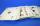How many times you can subtract the number 4 from the number 64?
• Identity123456789 = 100 Use only three plus or minus characters to correct previous identity/equation.
• CirclesHow many different circles is determined by 9 points at the plane, if 6 of them lie in a straight line?
• Classroom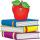In a classroom are 30 students, boys are four times more than girls. How many boys are in the class?
• Mom and daughter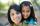Mom is 30 years older than the daughter. What is the age difference between them in 35 years?
• BracketsPlace one pair of brackets in expression 20-16 + 10 - 4 - 2 so that the result will be 0.
• RootThe root of the equation ? is (equal or greater or less than zero)? ?
• It is rectangular?Size of two of the angles in a triangle are: α=110°, β=40°. Is it a right triangle?
• Sparrow ball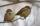On the sparrows ball were males and females. There were 170 sparrows. Males was 20 more than females. How many sparrow female was at a ball?
• Buffet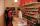In the school buffet were 30 children. 21 children bought sandwiches and 15 children bought lemonade. Four children did not buy anything. How many children bought lemonade and sandwiche?
• Television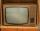When we add to the difference between the numbers 1704 and 654 the difference numbers 14920 and 14042, we get a year in which television was invented. How many years people known the TV?
• Landlord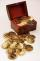Landlord had 49 ducats more than Jurošík. How many ducats Jurošík steal landlord if the Jurošík now 5 ducats more?
• Katy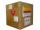Kate paid for the package on delivery of four meters textile 688 CZK. What is the price of one meter textile when shipping and handling amounted to 48 CZK?
• Cake fractions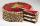Thomas ate 1/3 of cake, Bohouš of the rest of the cake ate 2/5. What fraction of cake left over for others?
• Six-eightsSix-eights of the one hundred pupils joined the Math Glee club. If the members of the Math Glee club were grouped into three, how many members were in each group?
• Temperature calculus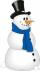Calculate the percentage of temperature increase if changed from 18°C to 33°C?
• Three numbersFind three numbers so that the second number is 4 times greater than the first and the third is lower by 5 than the second number. Their sum is 67.
• Plums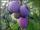In the bag was to total 136 plums. Igor took 3 plums and Mary took 4/7 from the rest. How many plums remained in the bag?
• Flowers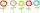The flower has six flowers, and each flower has a number. These are the numbers: 20,40,39,28,8,9. What number will be in the middle of the flower so that the numbers come from the flowers when we subtract and add?Add marks (+, -, *, /, brackets) to fullfill equations 1 3 6 5 = 10 This is for the 4th grade of the primary school - with no negative numbers yet